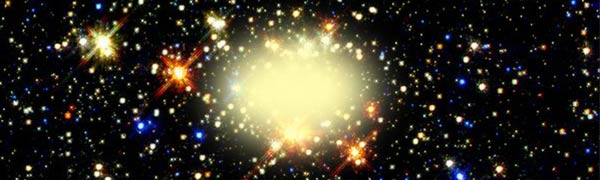# Space Is Full Of Hot GasA hot, spherically symmetric cloud of an ideal gas ($H_2$ for example) is in intergalactic space. The gas is in equilibrium and the density $\rho(r)$ of the gas is given by $\rho(r)=\rho_0r^{-2}$. What is the ratio of the temperature at a distance $r_0$ to the temperature at a distance $2r_0$?

×